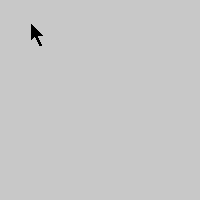# Random Walkers

example processing animation arraylist random-walker generative-art emergence

This example takes the previous Random Walker example and uses an `ArrayList` instead of an array.

``````ArrayList<RandomWalker> randomWalkers = new ArrayList<RandomWalker>();

void setup() {
size(200, 200);

background(200);

noSmooth();
frameRate(1000);
}

void draw() {
for (RandomWalker rw : randomWalkers) {
rw.step();
rw.draw();
}
}

void mouseDragged() {
}

class RandomWalker {
float x = mouseX;
float y = mouseY;
float r = random(256);
float g = random(256);
float b = random(256);

void step() {

x += random(-1, 1);
y += random(-1, 1);

if (x < 0) {
x = width;
}
if (x > width) {
x = 0;
}

if (y < 0) {
y = height;
}
if (y > height) {
y = 0;
}
}

void draw() {
stroke(r, g, b);
point(x, y);
}
}
``````Code Editor

See the Pen by Happy Coding (@KevinWorkman) on CodePen.

## Tweak Ideas

• Make it so the random walkers die when they exit the screen by removing them from the `ArrayList`.
• Make it so the random walkers die after a certain number of steps.
• Base the movement direction of each random walker off the direction the mouse is moving when the user clicks.